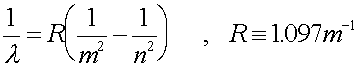Examples for atomic physics

Example #1

Problem:

a.) What is the energy of a photon emitted as an electron moves from the n = 5 to the n = 2 level in hydrogen?

Solution:

The energy of each level goes as (13.6 eV)/n2. The difference between the n = 5 and n = 2 values is the

energy of the photon's energy.

6.256 eV

b.) What is the wavelength of such a photon?

Solution:

Change the energy to Joules, then use E = hf to get the frequency. Then get the wavelength. OR use the formula:199 nm

c.) What is the energy of a photon in the same transition is carbon (Z=6)?

Solution:

The energy scales as Z2, so multiply the answer in part a by 36.

225 eV

Example #2

Problem:

a.) How many electrons are in an atom specified by 1s2,2s2,2p6,3s2,3p4?

Solution:

Count up the numbers in the superscripts.

16

b.) Write the spectroscopic notation for a neutral gold (Au) atom? See periodic table.

Solution:

See that a gold atom should have 79 electrons by looking at the periodic table. Then count up:

1s2,2s2,2p6,3s2,3p6,3d10,4s2,4p6,4d10,4f14,5s2,5p6,5d10,5f1

Atomic physics' index